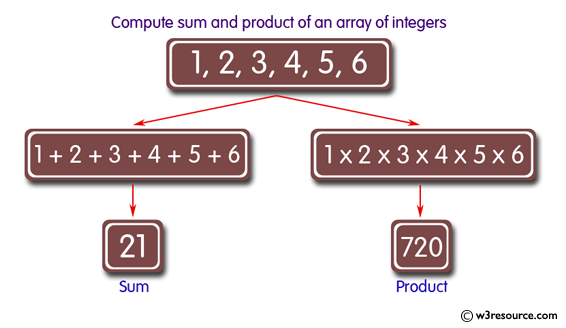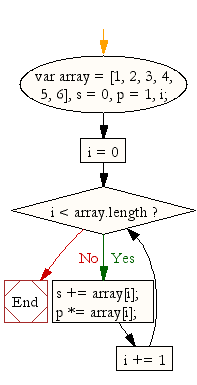# JavaScript: Compute the sum and product of an array of integers

## JavaScript Array: Exercise-12 with Solution

Write a JavaScript program to compute the sum and product of an array of integers.

Pictorial Presentation:Sample Solution:

HTML Code:

``````<!DOCTYPE html>
<html>
<meta charset=utf-8 />
<title>Compute the sum and product of an array of integers</title>
<body>
</body>
</html>
```
```

JavaScript Code:

``````var array = [1, 2, 3, 4, 5, 6],
s = 0,
p = 1,
i;
for (i = 0; i < array.length; i += 1)
{
s += array[i];
p *= array[i];
}
console.log('Sum : '+s + ' Product :  ' +p);
```
```

Sample Output:

```Sum : 21 Product :  720
```

Flowchart:ES6 Version:

``````const array = [1, 2, 3, 4, 5, 6];
let s = 0;
let p = 1;
let i;
for (i = 0; i < array.length; i += 1)
{
s += array[i];
p *= array[i];
}
console.log(`Sum : \${s} Product :  \${p}`);
``````

Live Demo:

See the Pen avaScript - sum and product of an array of integers- array-ex- 12 by w3resource (@w3resource) on CodePen.

Improve this sample solution and post your code through Disqus

What is the difficulty level of this exercise?

Test your Programming skills with w3resource's quiz.

﻿

## JavaScript: Tips of the Day

Converts a string to title case

Example:

```const toTitleCase = str =>
str
.match(/[A-Z]{2,}(?=[A-Z][a-z]+[0-9]*|\b)|[A-Z]?[a-z]+[0-9]*|[A-Z]|[0-9]+/g)
.map(x => x.charAt(0).toUpperCase() + x.slice(1))
.join(' ');
console.log(toTitleCase('some_database_field_name')); // 'Some Database Field Name'
console.log(toTitleCase('Some label that needs to be title-cased')); // 'Some Label That Needs To Be Title Cased'
console.log(toTitleCase('some-package-name')); // 'Some Package Name'
console.log(toTitleCase('some-mixed_string with spaces_underscores-and-hyphens')); // 'Some Mixed String With Spaces Underscores And Hyphens'
```

Output:

```"Some Database Field Name"
"Some Label That Needs To Be Title Cased"
"Some Package Name"
"Some Mixed String With Spaces Underscores And Hyphens"
```